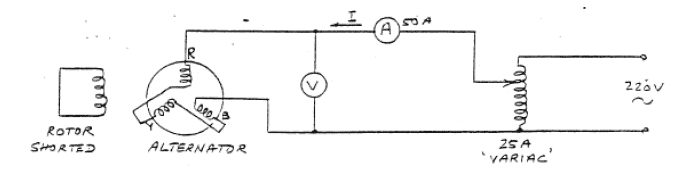# Negative and zero sequence impedance of alternator

TheRedDevil18
To measure negative sequence impedance of an alternator, we short the white and blue phases, measure the current and then divide it by the voltage of the red phase. Then to measure zero sequence impedance, we short the rotor and connect the stator in open delta and inject a current.

My question is, how or why does it give us the negative and zero sequence impedance ?, I don't quite understand the method

Staff Emeritus
To measure negative sequence impedance of an alternator, we short the white and blue phases, measure the current and then divide it by the voltage of the red phase. Then to measure zero sequence impedance, we short the rotor and connect the stator in open delta and inject a current.

My question is, how or why does it give us the negative and zero sequence impedance ?, I don't quite understand the method

Think of the underlying reality. Positive, negative and zero sequences are merely mathematical tricks used to analyze unbalanced positive three phase networks. By cleverly creating unbalance unbalances in your test methods, you isolate the components.

But what do those negative and zero sequence values represent? Unbalances.

You can combine P, N, Z components in ways to represent other kinds of unbalance that you did not test.

Homework Helper
Gold Member
Then to measure zero sequence impedance, we short the rotor and connect the stator in open delta and inject a current.
Zero sequence impedance is nothing but the leakage impedance of the alternator. You can connect the stator phases in series or parallel such that instantaneous current through each of them is in the same direction. Formula for each case will be different, but it should ultimately give the same value. This is how we did it in lab. Here, the magnetic field in the air gap is zero because all the windings carry the same current, which gives rise to three equal fluxes 120° apart in space.
I don't know the method you described. Could you provide a circuit diagram?

About the negative sequence impedance procedure- You are creating a line-line fault. So the fault current will consist of both +ve and -ve sequence currents (no zero sequence). I will think about how the separation of the components is done and post again later.

Meanwhile, here is another way of measuring the negative sequence impedance:
Short the rotor and give 3 phase supply to the stator. Rotate the rotor using some prime mover in the opposite direction to that of the stator mmf. Now, Vph/Iph gives the negative sequence impedance (reactance) of the alternator. This method is more intuitive and easy to understand as per the definition of -ve sequence current.

Homework Helper
Gold Member
To measure negative sequence impedance of an alternator, we short the white and blue phases, measure the current and then divide it by the voltage of the red phase
Actually, we measure the line voltage VRB and divide it by √3*I to get the negative sequence impedance.

After playing with some equations for some time, I managed to prove how this procedure gives the negative sequence impedance.
Not a single book or lab manual I referred so far gives any explanation about this method. But it turns out that this is a clever method designed from the equations for LL fault.

You are shorting the white (you sure it's white and not yellow?) and blue phases.

Find an expression for negative sequence component of voltage V2 in terms of line voltages. It comes out to be,
V2=(VR-VB)/3.

Now find an expression for fault current (i.e. -IB or IW) in terms of negative sequence current I2.
This gives
I2=IW/√3.

∴Z2=V2/I2
=(VRB)/(√3IW)

..which is what we get from the given arrangement.

Last edited:
•Asymptotic, TheRedDevil18 and anorlunda
TheRedDevil18
I don't know the method you described. Could you provide a circuit diagram?

Zero sequence impedance measurement of alternator:Xo = V/3I

I've read that for delta connections, the zero sequence currents are zero on the lines but are present within the phases. Not sure if that applies here

•Asymptotic
Homework Helper
Gold Member
Zero sequence impedance measurement of alternator:
The stator phases are connected in series (I don't know if that is called 'open delta') in the diagram you posted.
I've read that for delta connections, the zero sequence currents are zero on the lines but are present within the phases. Not sure if that applies here
Zero sequence currents do not create a rotating magnetic field. So the reactance offered is largely due to the leakage reactance of the windings. Connecting the windings in series gives rise to three currents equal in magnitude and in phase. These currents are 120 apart in space, so there is no rotating field. To calculate leakage reactance per phase, we use X0=V/3I. If the stator phases were conneted in parallel, it would be X0=V/Iphase.

Last edited:
•jim hardy, Asymptotic and TheRedDevil18